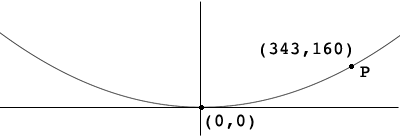SEARCH HOMEMath Central Quandaries & QueriesQuestion from Monica:A parabolic curve on a bridge how do i find the formula for my parabola with the provided information: the golden gate bridge has a parabola (343,160) = coordinate (0,0)= vertexHi Monica,

The parabola is symmetric around the Y-axis, has vertex at the origin and a point on the parabola has a positive Y-coordinate so I know that it opens upward and its equation is of the form $y = a \; x^{2},$ where $a$ is positive.Use that fact that the point $(343,160)$ lies on the curve to determine that value of $a.$

PennyMath Central is supported by the University of Regina and The Pacific Institute for the Mathematical Sciences.